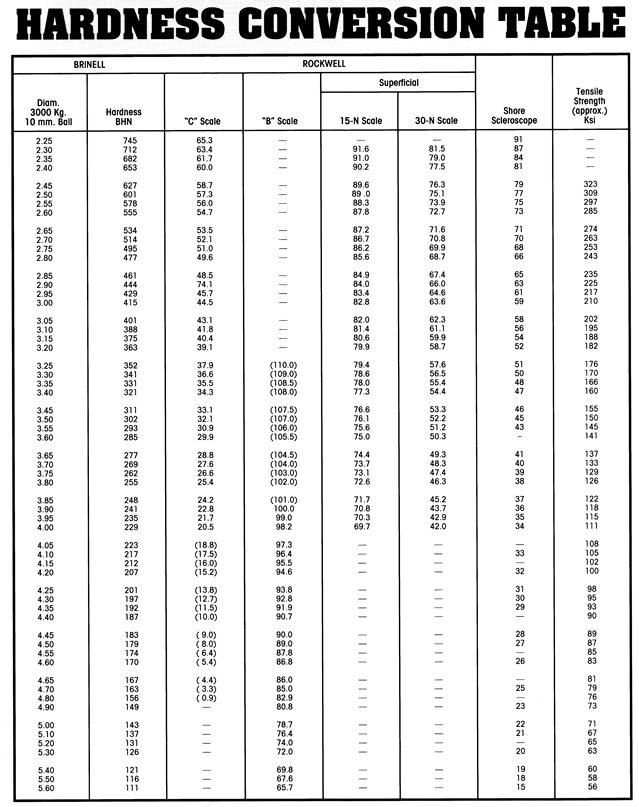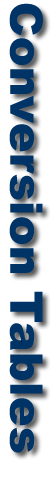Wellington Alloys LLC | P.O. Box 250298 | Franklin, MI. 48025 | Office 248-737-4216 | Fax 248-737-4215 |  VISA • Mastercard • AMEX
 Helpful Information Rounds Flats Hex and Squares Metric Conversions Hardness Conversions To find the circumference of a circle: Multiply the radius by 6.2832, or Multiply the diameter by 3.1416, or Multiply the square root of the area by 3.5449 To find the radius of a circle: Multiply the diameter by .5, or Multiply the circumference by. 15915, or Multiply the square root of the area by .5641 To find the diameter of a circle: Multiply the radius by 2, or Multiply the circumference by .31831, or Multiply the square root of the area by 1.1284 To find the area of a circle: Multiply the square of the radius by 3.1416, or Multiply the square of the diameter by .7854, or Multiply the square of the circumference by .07958 To find the area of a hexagon: Multiply the square of the distance across b the area of the inscribed circle by 1.1027 To find the area of an octagon: Auftiply the square of the distance across by .82843, or Multiply the area of the inscribed circle by 1.0548 To find the area of a rectangle: ultiptythe length by the width ' To find the area of a triangle: Multiply the base by one-half the perpendicular height 86603, or Multiply To find the side of an inscribed square: Multiply the diameter by .7071, or Multiply the circumference by :2251 To find the side of an equal square: Multiply the diameter by.8862 To find the diameter of the circumscribing circle of a square: Multiply a side by 1.4142 To find the circumference of the circumscribing circle of a square: Multiply a side by 4.443 To find the cubic contents of a cone: Multiply the area of the base by one-third the altitude To find the area of an ellipse: Multiply the product of its axes by :785d To find the area of 4 parallelogram: Multiply the base times the perpendicular height To find the volume of a parallelogram: Multiply the area of cross section times the length To find the area of a cylinder: Multiply the length times the circumference of plus the area of both ends To find the volume of a cylinder: Multiply the area of the base by the perpendicular height To find the area of a sphere: Multiply the square of the diameter by 3.1416, or Multiply the diameter times the circumference To find the volume of a sphere: Multiply the cube of the diameter by .5236 To find the capacity of a tank in gallons: (All measurements must be reduced to inches For cylindrical tanks, multiply the length by the square of the diameter by .0034. For rectangular tanks, multiply the length by the width by the depth and divide by 231.' For elliptical tanks, multiply the length by the short diameter ' by the long diameter by .0034. ; To convert Brinell Hardness to tensile strength: Divide the Brinell Hardness number by two to get the approxi­mate tensile strength in thousands of pounds per square inch. Example: Assume Brinetl Hardness of 248. 248 = 2 = 124,000 p.s.i. (approx. tensile strength). nversely, drop the last three figures of the tensile strength and multiplying by two to get the approximate Brinell Hardness number. Example: Assume tensile strength of 122,000 p.s.i. 122 X 2 = 244 (approximate Brinell Hardness). estimate the weight of a round steel bar: Multiply the diameter by 4, square the product, and divide by 6. The result is the approximate weight in pounds per foot of length. To estimate the weight of a square steel bar: Square the size, add a zero and divide by 3. The result is the approximate weight in pounds per foot of length. To estimate the weight of a flat steel bar: Multiply the width by the thickness, add a zero and divide by 3. The result is the approximate weight in pounds per foot of length. Manganese (Mn) Manganese is a deoxidizer and degasifier. It also reacts with ulphur to improve forgeability. Manganese increases tensile trength, hardness, hardenability, resistance to wear and the rate of carbon penetration in carburizing. It also decreases the tendency toward scaling and distortion. Molybdenum (Mo) Molybdenum increases strength, toughness, hardness, and hardenability as well as creep resistance and strength at ele­vated temperatures. It improves machinability, corrosion resis­ nce and intensifies the effects of the other alloying elements. In hot-work steels, molybdenum increases redhardness proper­ties. Nickel (Ni) Nickel increases strength and hardness with no loss of ductility and toughness. It also increases resistance to corrosion and scaling at elevated temperatures when introduced in suitable quantities in high chromium stainless steels. Phosphorus (P) Phosphorus increases strength and hardness and improves machinability. However, it adds brittleness or cold-shortness to steel. Silicon (Si) Silicon is a deoxidizer and degasifier. Also, it increases the ten­sile and yield strengths, forgeabilitv, hardness and magnetic permeability. Sulphur (S) Sulphur improves machinability in free-cutting steels. It decreases weldability, ductility and impact strength. Also, the addition of sulphur without sufficient manganese produces brit­tleness at red heat. Tantalum (To) Tantalum is used as a stabilizing element in stainless steels. It has a high affinity for carbon and forms carbides which are uniformly dispersed throughout the steel, thus prevent ing localized depletion of carbon at grain boundaries. Titanium (Ti) Titanium, like tantalum and columbium, is added to stainless steels to make them immune to harmful carbide precipitation. Tungsten (W) Tungsten increases strength, toughness and hardness. At ele­vated temperatures tungsten steels have superior yet working characteristics and greater cutting efficiency. Vanadium (V) Vanadium increases strength, hardness, and impact resis­tance. By retarding grain growth vanadium permits higher quenching temperatures. It also improves the red-hardness properties of high-speed metal cutting tools and intensifies the individual effects of other major elements. Content 2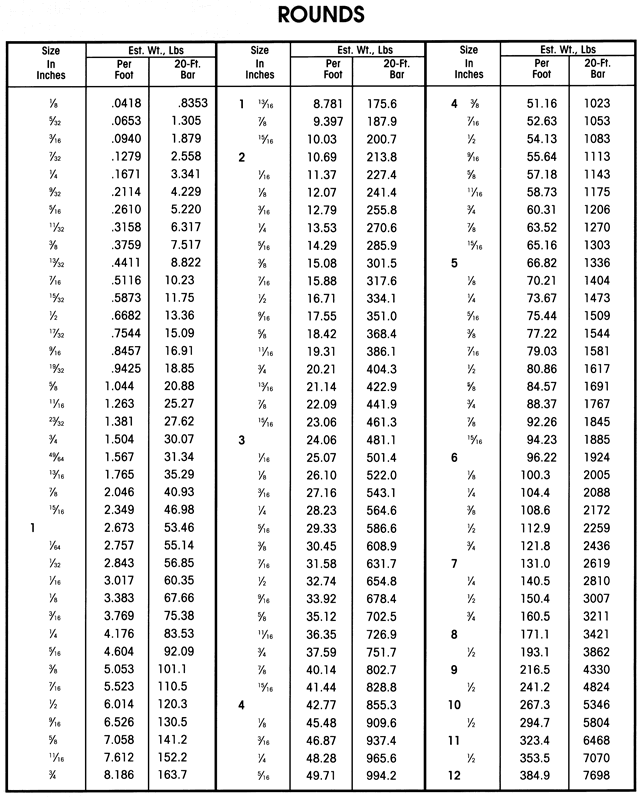Content 3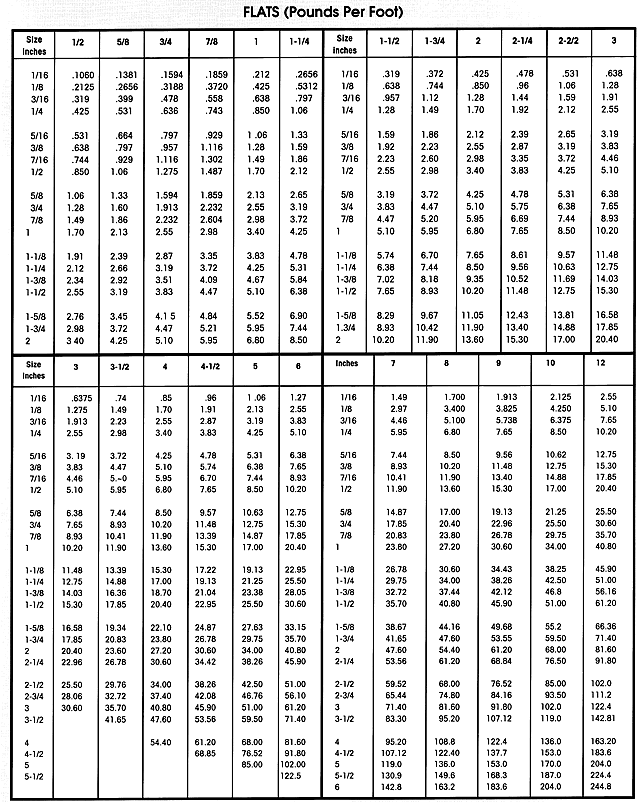Content 4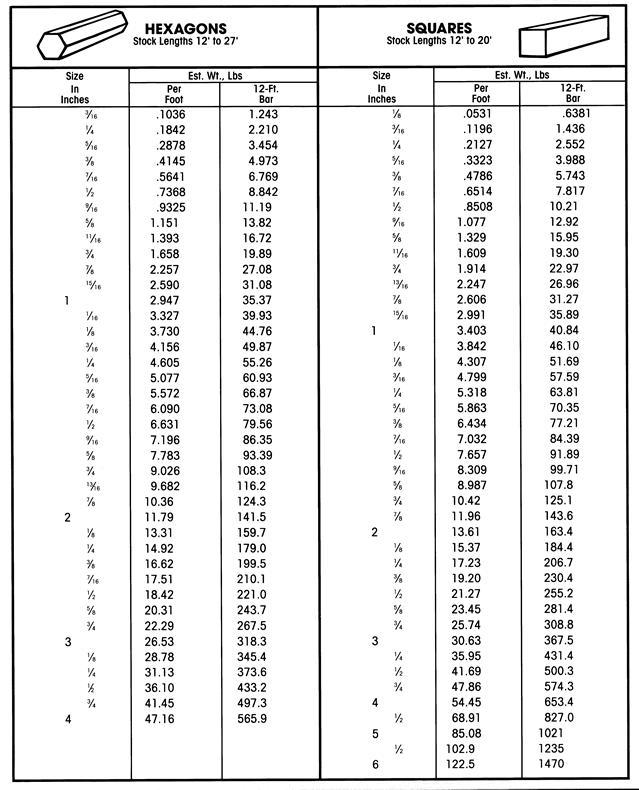Content 5Content 6